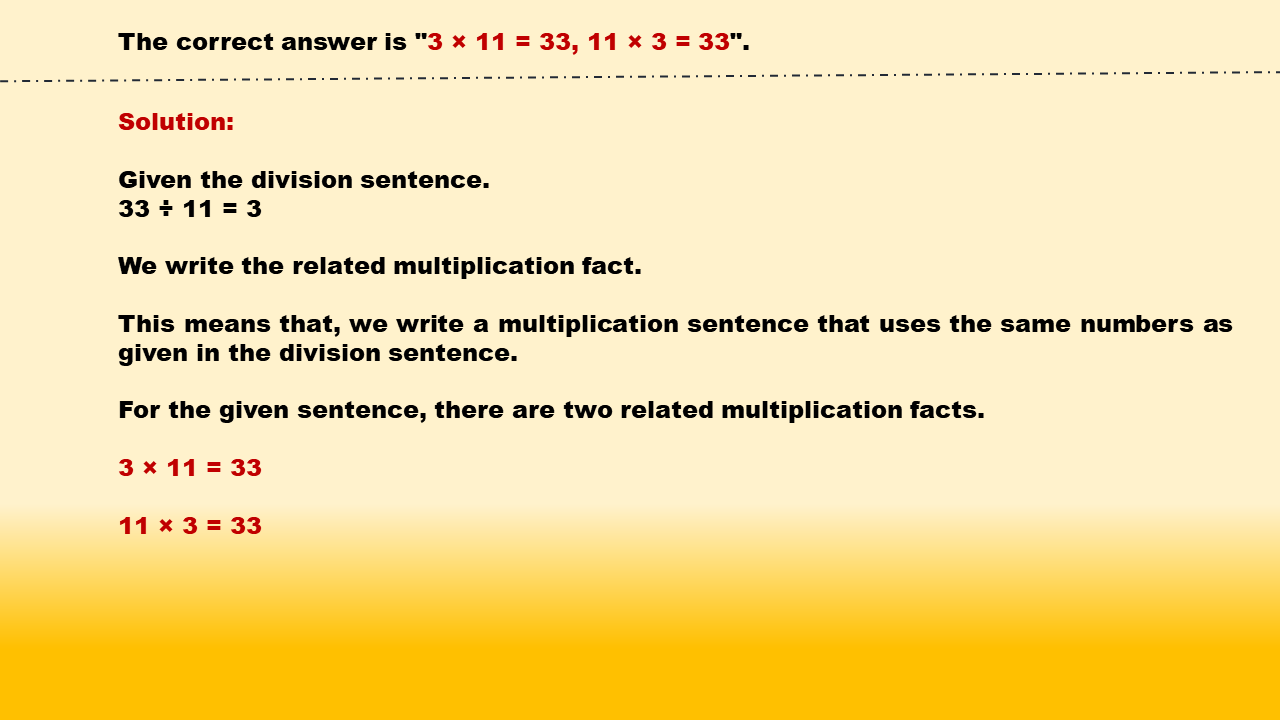1
visibility

Identify related multiplication facts for 33 ÷ 11 = 3.

• 3 × 12 = 36, 12 × 3 = 36

• 3 × 11 = 33, 11 × 3 = 33

• 3 × 5 = 15, 5 × 3 = 15

• 3 × 7 = 21, 7 × 3 = 21